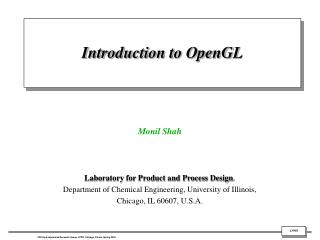DownloadDownload PresentationIntroduction to OpenGL

# Introduction to OpenGL

Télécharger la présentation## Introduction to OpenGL

- - - - - - - - - - - - - - - - - - - - - - - - - - - E N D - - - - - - - - - - - - - - - - - - - - - - - - - - -
##### Presentation Transcript

1. Introduction to OpenGL Monil Shah Laboratory for Product and Process Design, Department of Chemical Engineering, University of Illinois, Chicago, IL 60607, U.S.A.

2. Motivation • Graphics rendering API (application programming interface) • high-quality color images composed of geometric and image primitives • window system independent • operating system independent • What can it do for us??? • GLU (OpenGL Utility Library), GLUT (OpenGL Utility Toolkit) • portable windowing API • Geometric primitives : points, lines and polygons • Rendering depends on state : colors, materials, light sources, etc.

3. Application Structure • Configure and open window • Initialize OpenGL state • Register input callback functions • render • resize • input: keyboard, mouse, etc. • Enter event processing loop

4. Creating an OpenGL window

5. Include the header files #include <GL/gl.h> #include <GL/glut.h> • Display function void display (void) { glClearColor (0.0,0.0,0.0,1.0); //clear the color of the window glClear (GL_COLOR_BUFFER_BIT); //Clear the Color Buffer (more buffers later on) glLoadIdentity(); //load the Identity Matrix gluLookAt (0.0, 0.0, 5.0, 0.0, 0.0, 0.0, 0.0, 1.0, 0.0); //set the view glFlush(); //flush it all to the screen, submit for hardware execution } • Main • int main (int argc, char **argv) { • glutInit (&argc, argv); //initialize the program. • glutInitDisplayMode (GLUT_SINGLE); //set up a basic display buffer (only singular for now) • glutInitWindowSize (500, 500); //set the width and height of the window • glutInitWindowPosition (100, 100); //set the position of the window • glutCreateWindow ("A basic OpenGL Window"); //set the caption for the window • glutDisplayFunc (display); //call the display function to draw our world • glutMainLoop (); //initialize the OpenGL loop cycle • return 0; • }

6. Creating a 3D Sphere

7. glPushMatrix(); glMaterialfv(GL_FRONT,GL_DIFFUSE,color); glutSolidSphere(1,20,20); Or glutWireSphere(1,20,20); radius : for sphere slices : The number of subdivisions around the Z axis (similar to lines of longitude). stacks :The number of subdivisions along the Z axis (similar to lines of latitude). glPopMatrix(); glutSwapBuffers();

8. Decreasing slices and stacks

9. Changing the View

11. Lighting effect • //Specifies a symbolic value representing a shading technique. • glShadeModel(GL_SMOOTH); • /*If enabled, do depth comparisons and update the depth buffer. glEnable(GL_DEPTH_TEST); • /*If enabled, use the current lighting parame-parameters to compute the vertex color or index. • Otherwise, simply associate the current color index with each vertex.*/ • glEnable(GL_LIGHTING); • // lighting effect • GLfloat lightZeroPosition[] = {10.,4.,10.,1.}; • GLfloat lightZeroColor[] = {0.8,0.8,0.8,1.0}; • glLightfv(GL_LIGHT0, GL_POSITION, lightZeroPosition); • glLightfv(GL_LIGHT0, GL_DIFFUSE, lightZeroColor); • glLightf(GL_LIGHT0, GL_CONSTANT_ATTENUATION, 0.1); • glLightf(GL_LIGHT0, GL_LINEAR_ATTENUATION, 0.05); • glEnable(GL_LIGHT0);

12. The Ortho function glOrtho (-alpha , alpha , -alpha , alpha, -alpha , alpha);

13. A simple pyramid grid from Gambit

14. Opening and reading the file struct point3D { GLdouble x,y,z; }; point3D inputGrid; int i = 0; int count; int j ; int initGrid() { //define a string const int MAX = 200; char str[MAX]; // read file ifstream infile("D:\\pyramidgrid.txt");

15. while(!infile.eof()) { // reading line by line infile.getline(str,MAX); //if (str != NULL) //{cout << endl <<str;} int j = 0 ; char * pch; pch = strtok (str," "); // removing space and converting ascii to float, double while (pch != NULL) { if (j == 0) { // loading values into an array inputGrid [i].x = atof (pch); }

16. if (j == 1) { inputGrid [i].y = atof (pch); } if (j == 2) { inputGrid [i].z = atof(pch); } //cout<< endl<< pch<< endl; pch = strtok (NULL, " "); j++; } i++; }

17. Output

18. Display grid